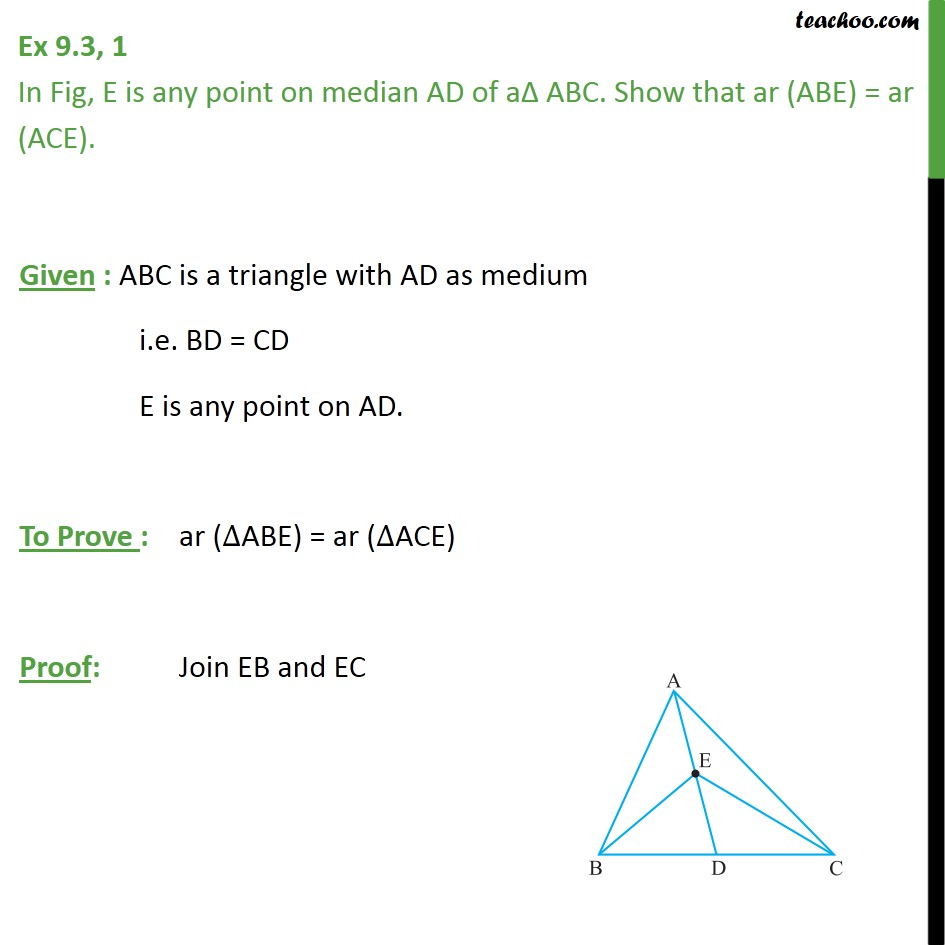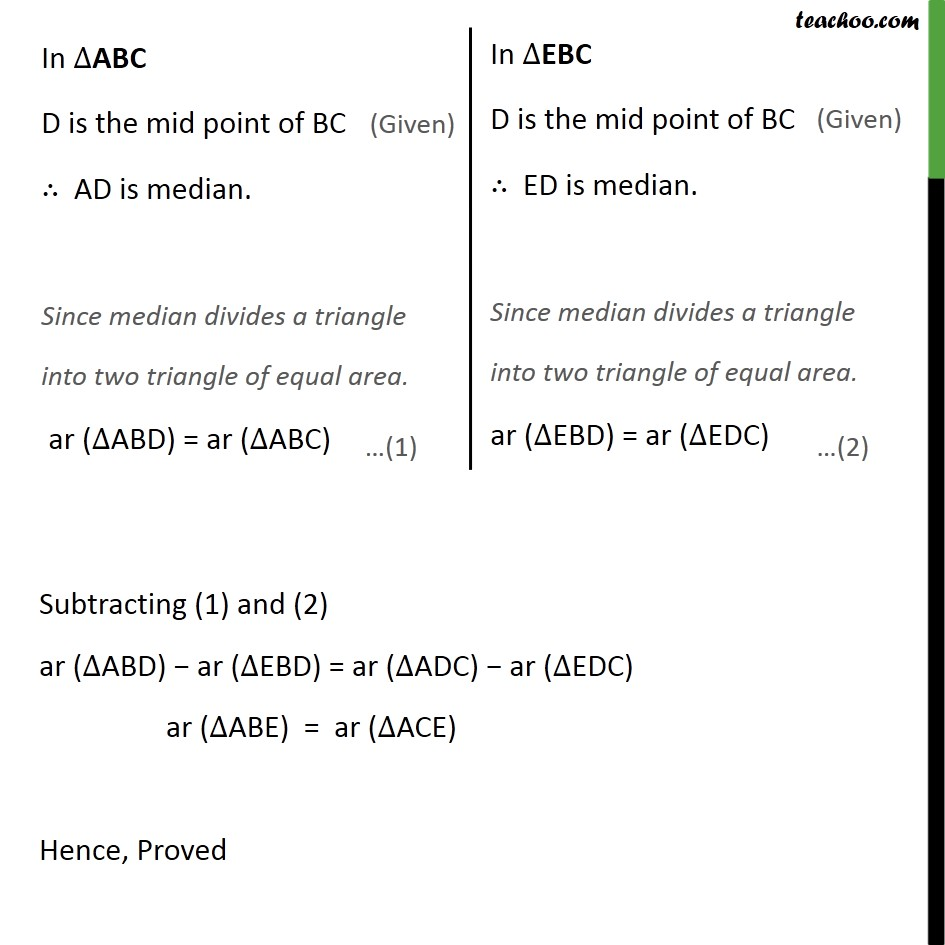Area of Triangles

Areas of Parallelograms and Triangles
Serial order wiseLearn in your speed, with individual attention - Teachoo Maths 1-on-1 Class

### Transcript

Question 1 In Fig, E is any point on median AD of a ABC. Show that ar (ABE) = ar (ACE). Given : ABC is a triangle with AD as medium i.e. BD = CD E is any point on AD. To Prove : ar ( ABE) = ar ( ACE) Proof: Join EB and EC Subtracting (1) and (2) ar ( ABD) ar ( EBD) = ar ( ADC) ar ( EDC) ar ( ABE) = ar ( ACE) Hence, Proved